Ex 14.2

Chapter 14 Class 7 Symmetry
Serial order wise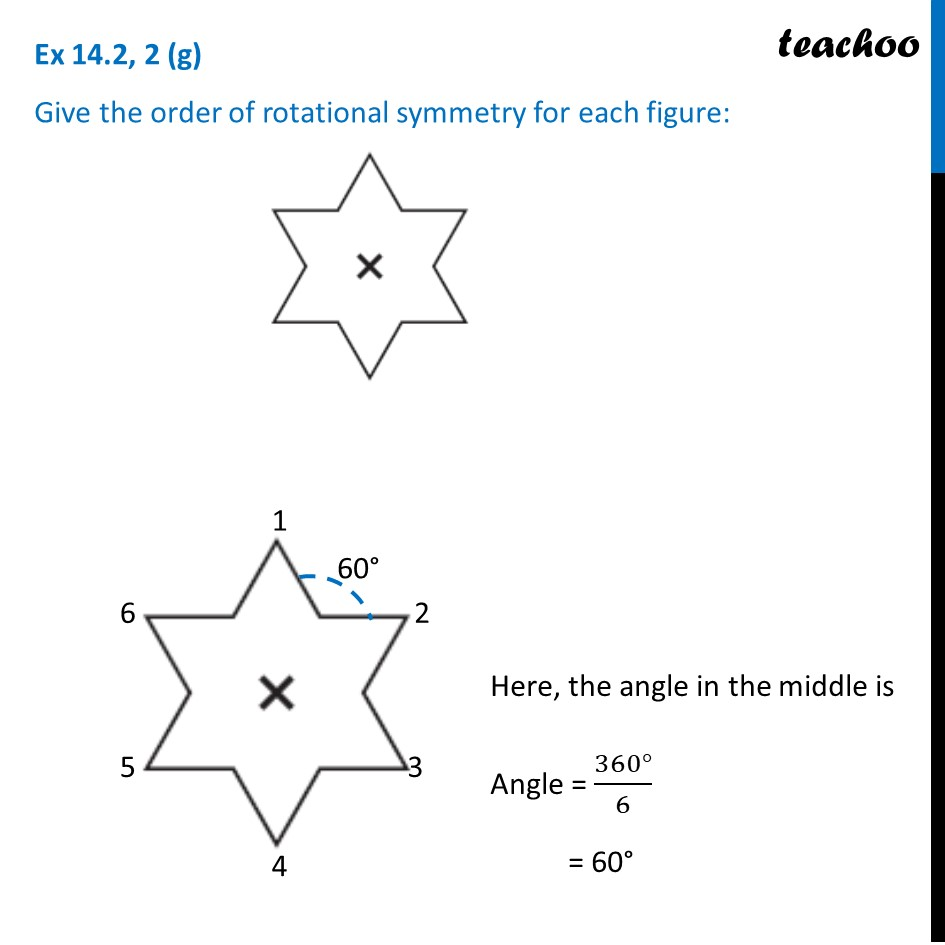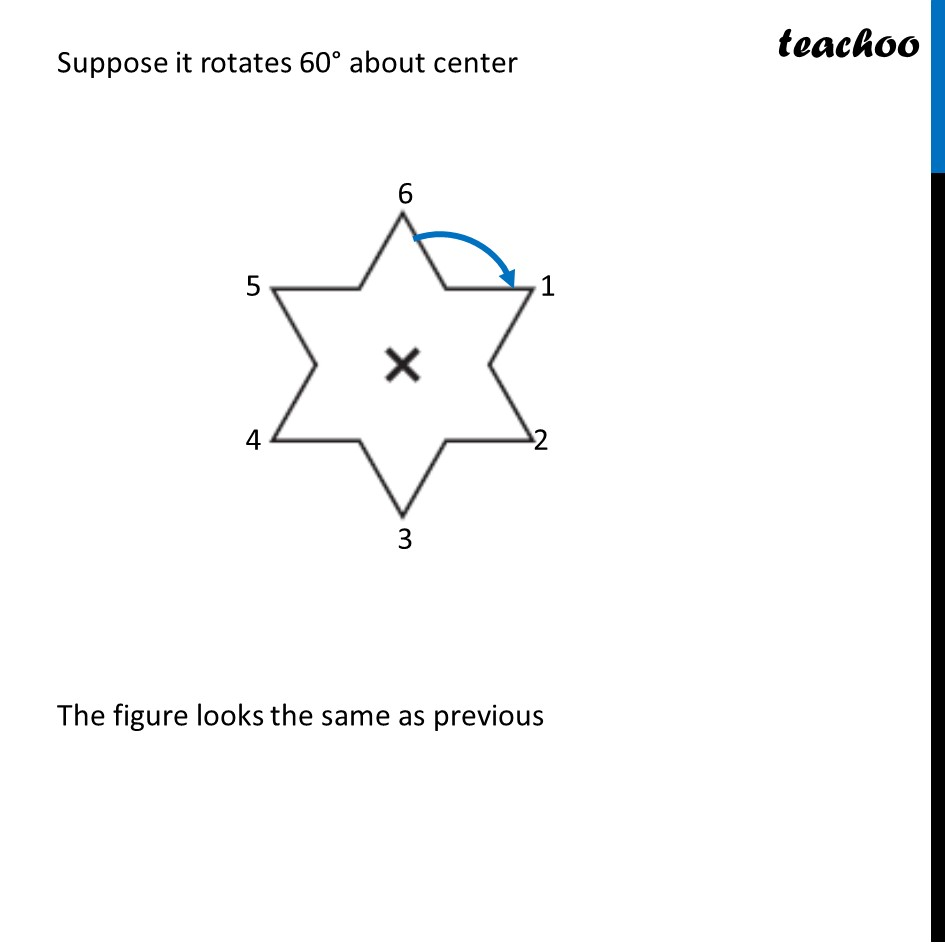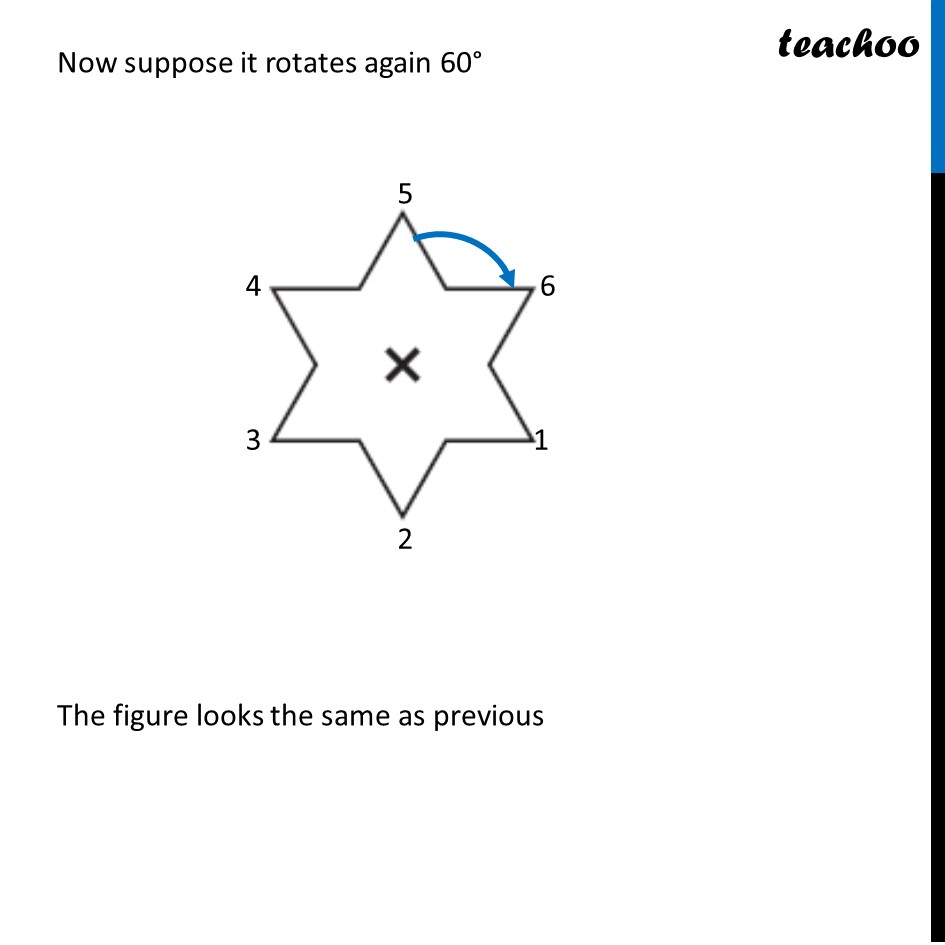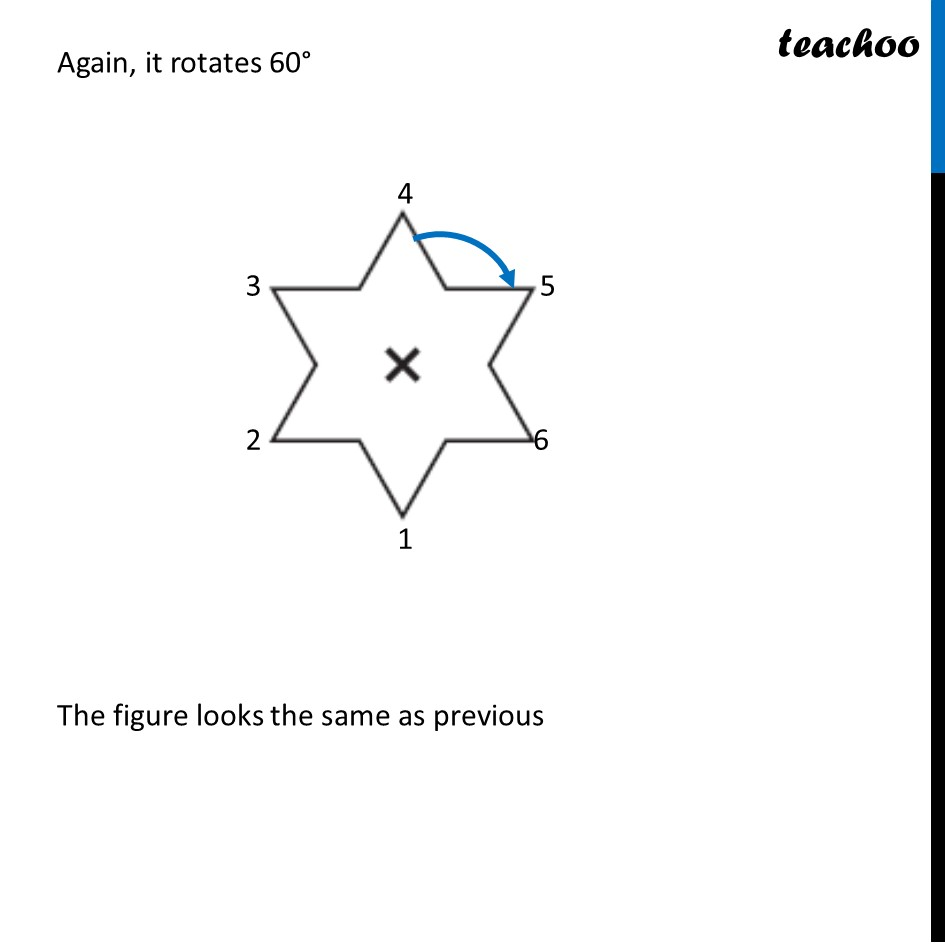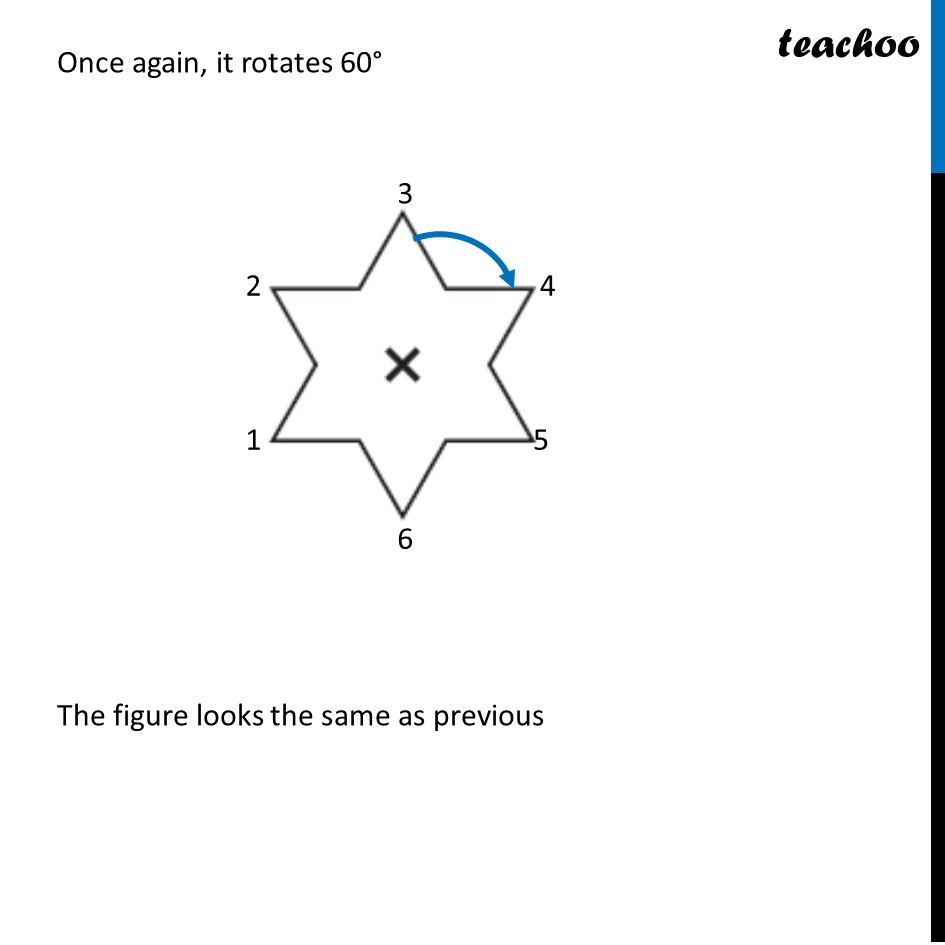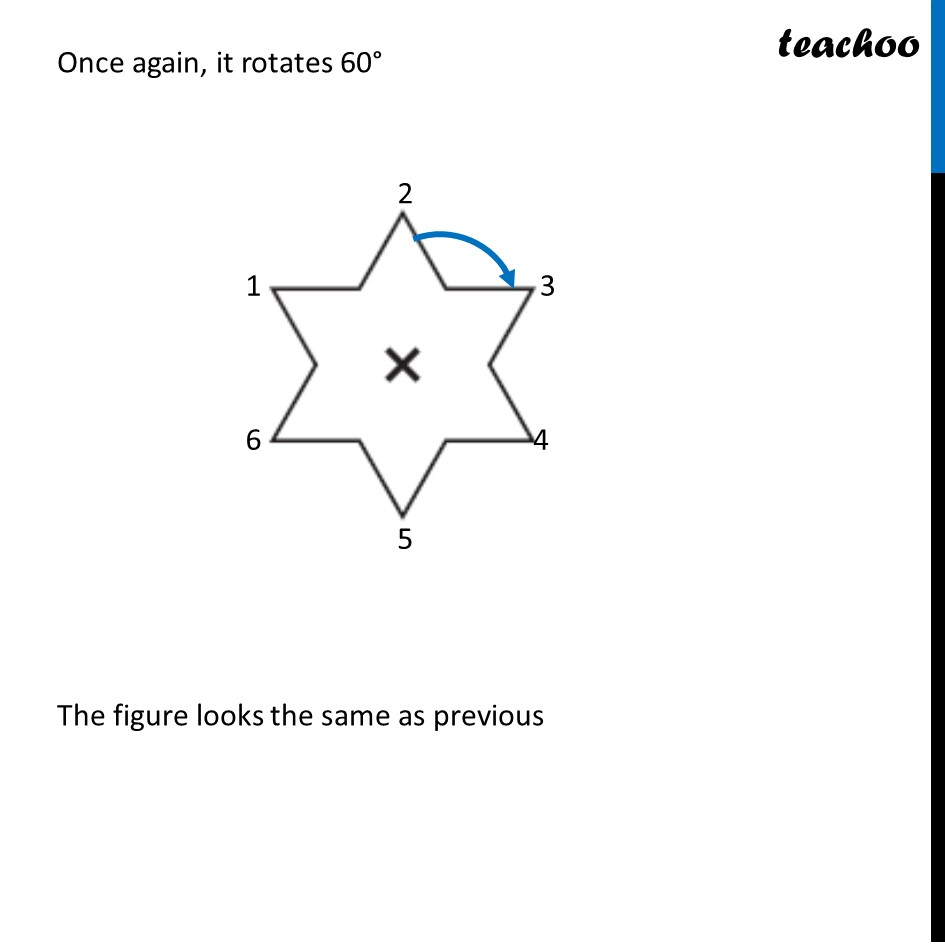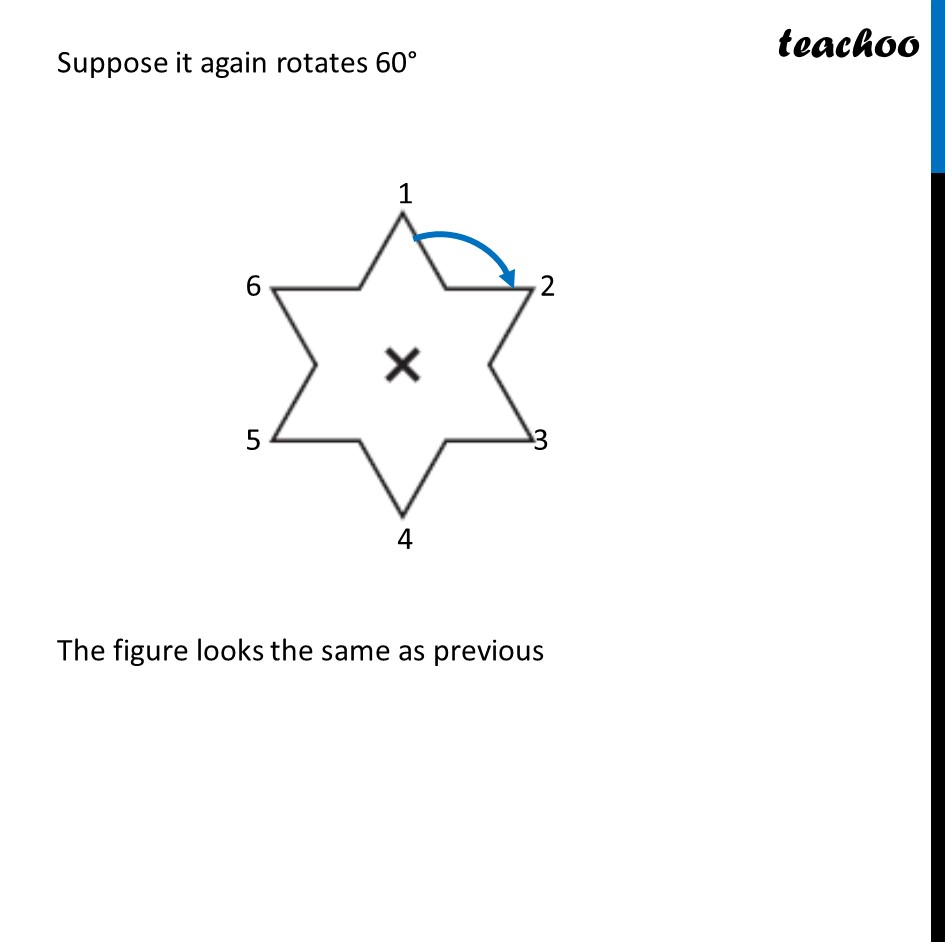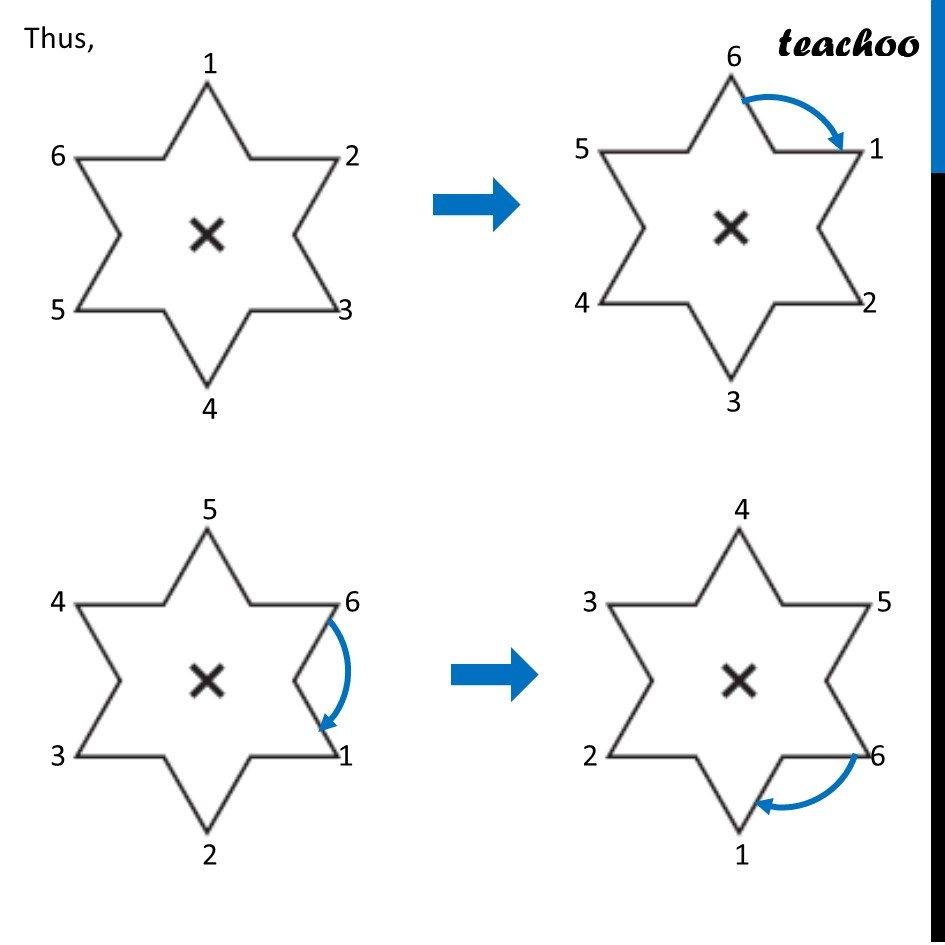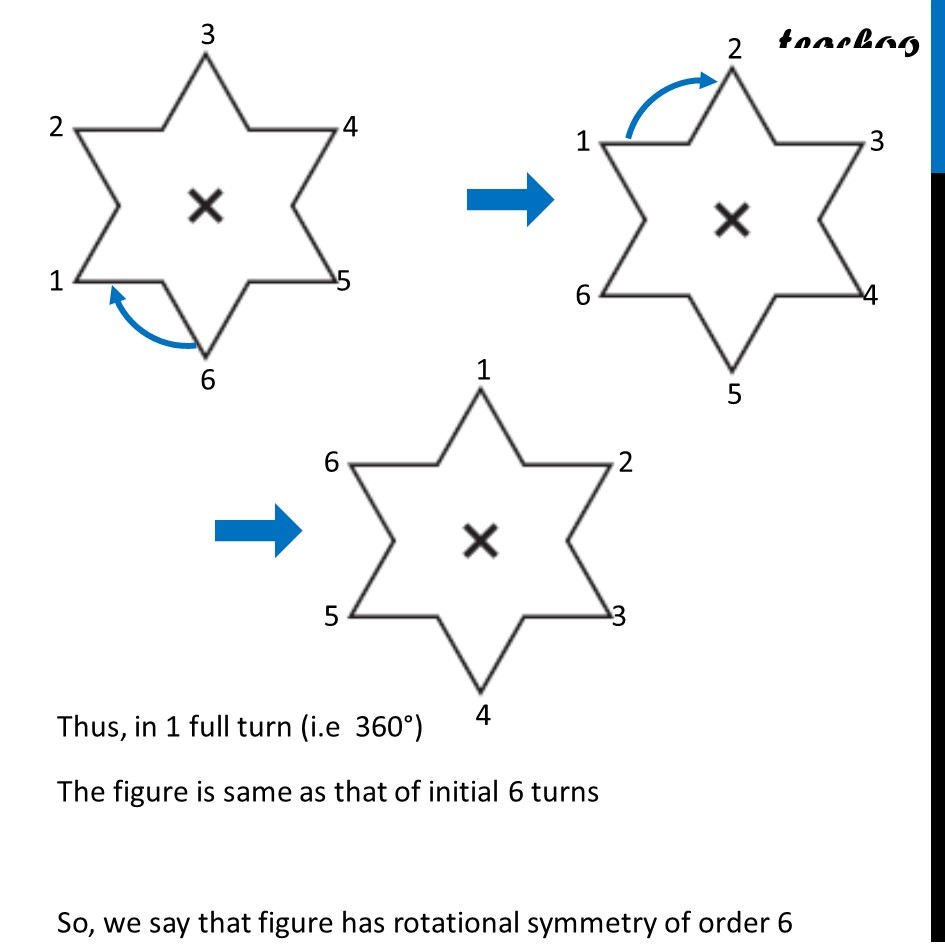Get live Maths 1-on-1 Classs - Class 6 to 12

### Transcript

Ex 14.2, 2 (g) Give the order of rotational symmetry for each figure: Here, the angle in the middle is Angle = (360°)/6 = 60° Suppose it rotates 60° about center The figure looks the same as previous Now suppose it rotates again 60° The figure looks the same as previous Now suppose it rotates again 60° The figure looks the same as previous Again, it rotates 60° The figure looks the same as previous Once again, it rotates 60° The figure looks the same as previous Once again, it rotates 60° The figure looks the same as previous Suppose it again rotates 60° The figure looks the same as previous Thus, Thus, in 1 full turn (i.e 360°) The figure is same as that of initial 6 turns So, we say that figure has rotational symmetry of order 6